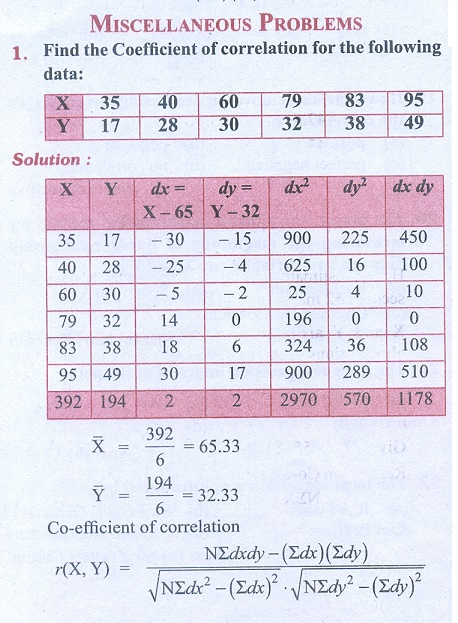Home | | Business Maths 11th std | Correlation and Regression analysis: Miscellaneous Problems

# Correlation and Regression analysis: Miscellaneous Problems

Business Mathematics and Statistics Book back answers and solution for Exercise questions - Statistics: Correlation and Regression analysisTags : Problem Questions with Answer, Solution | Statistics , 11th Business Mathematics and Statistics(EMS) : Chapter 9 : Correlation and Regression analysis
Study Material, Lecturing Notes, Assignment, Reference, Wiki description explanation, brief detail
11th Business Mathematics and Statistics(EMS) : Chapter 9 : Correlation and Regression analysis : Correlation and Regression analysis: Miscellaneous Problems | Problem Questions with Answer, Solution | Statistics